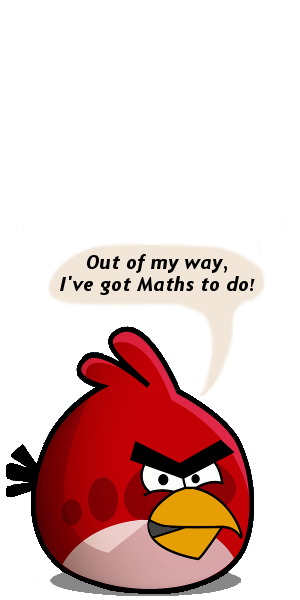# Year 9 Revision

## Practice Exams

Here are two exams that are the same length and difficulty as the final exams, with answers for each of the four sections: Algebra (including lines), Measurement, Number and Geometry.

Practice Exam #1     Answers Exam #1

Practice Exam #2     Answers Exam #2

## Number

Number Notes I and Number Notes II

Notes for the key skills in the topic.

### Basic Number Theory

Number Terms Cards

The terms and concepts you need to know. These are set up to be printed as flash cards.

Practice 1     Practice 2     Practice 3

Practice 4     Practice 5     Extension

Twenty mixed questions on one worksheet, with answers on a second sheet.

### Percentages and Fractions

Practice 1     Practice 2     Practice 3

Practice 4     Practice 5     Extension

Twenty mixed questions on one sheet, with answers on a second sheet.

### Ratios and Proportion

(Note: formal ratios are not part of Year 9, but it is useful to see them early.)

Practice 1     Practice 2     Practice 3

Twelve mixed questions on one sheet, with answers on a second sheet.

## Measurement

Measurement Notes

Notes on all the key skills, plus some higher level material.

### Units, Conversions, Rates

Practice 1     Practice 2     Practice 3

Practice 4     Practice 5     Extension

Twenty mixed questions on one sheet, with answers on a second sheet.

### Calculating Perimeter, Area and Volume

Practice 1     Practice 2     Practice 3

Practice 4     Practice 5     Extension

Perimeter, area, volume and surface area questions mixed on one worksheet, with answers on a second sheet.

## Algebra

Algebra Notes

There are more worksheets than fit comfortably here, so they are grouped on a separate Algebra Page.

Explanatory videos of almost all the topic are on the Videos Page.

## Patterns and Graphs (Algebra II)

Patterns and Graphing Notes

What you should be able to do. Note that the Videos Page has much of this information in video form.

This allows students to test their ability to draw lines from formulas and to write equations from drawn lines and patterns.

Practice 1     Practice 2     Practice 3

Practice 4     Practice 5     Extension

Patterns points and lines questions, with answers on a second sheet.

## Shapes and Angles (Geometry I)

Geometry Cards

Terms and concepts you need to know. These are set up to be printed as flash cards.

Practice 1     Practice 2     Practice 3

Practice 4     Practice 5     Extension

Geometry questions, with answers on a second sheet.

## Transformations (Geometry II)

Transformations Cards

The terms and concepts you need to know. These are set up to be printed as flash cards.

Reflection 1    Rotation 1    Translation 1

Reflection 2    Rotation 2    Translation 2

Exercises on the key parts of the topic, with answers on a second page.

Symmetry 1    Isometric 1    Enlargement 1

Symmetry 2    Isometric 2    Enlargement 2

Minor parts of the topic. (Enlargement is an extension topic at Year 9.)

## Statistics

Statistics Notes

The scope of the whole topic, in quite a lot of detail.

Statistics Cards

Terms and concepts you need to know. These are set up to be printed as flash cards.

Practice 1     Practice 2     Practice 3

Practice 4     Practice 5     Extension

Statistics practice questions, with answers on a second sheet.

## Probability

Probability Notes

The concepts for this year. (Note: this year is an introduction to several new concepts – especially probability trees – and while it is good if they are mastered, it is not vital yet.)

Practice 1     Practice 2     Practice 3

Practice 4     Practice 5     Extension

Probability practice questions, with answers on a second sheet.